Prime Factorization 1
Set 1 (10 Questions)
Determine the prime factors of all numbers through 50 and write the numbers as the product of their prime factors by using exponents to show multiples of a factor.
From Mr. Anker Tests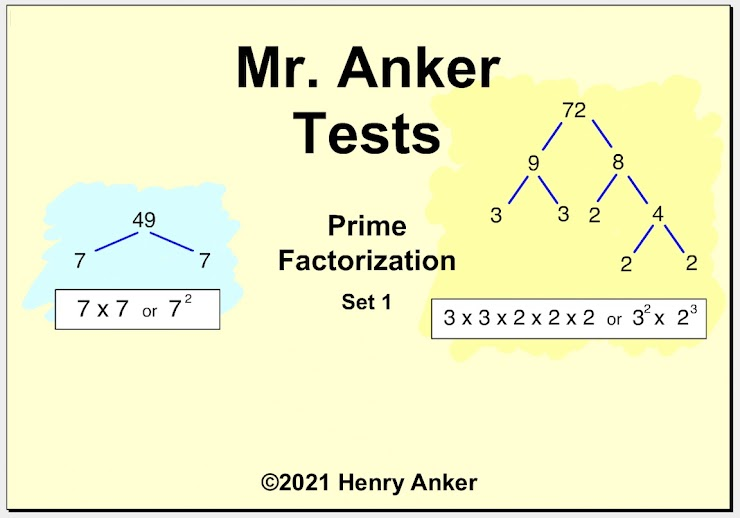Prime Factorization Help Slide 1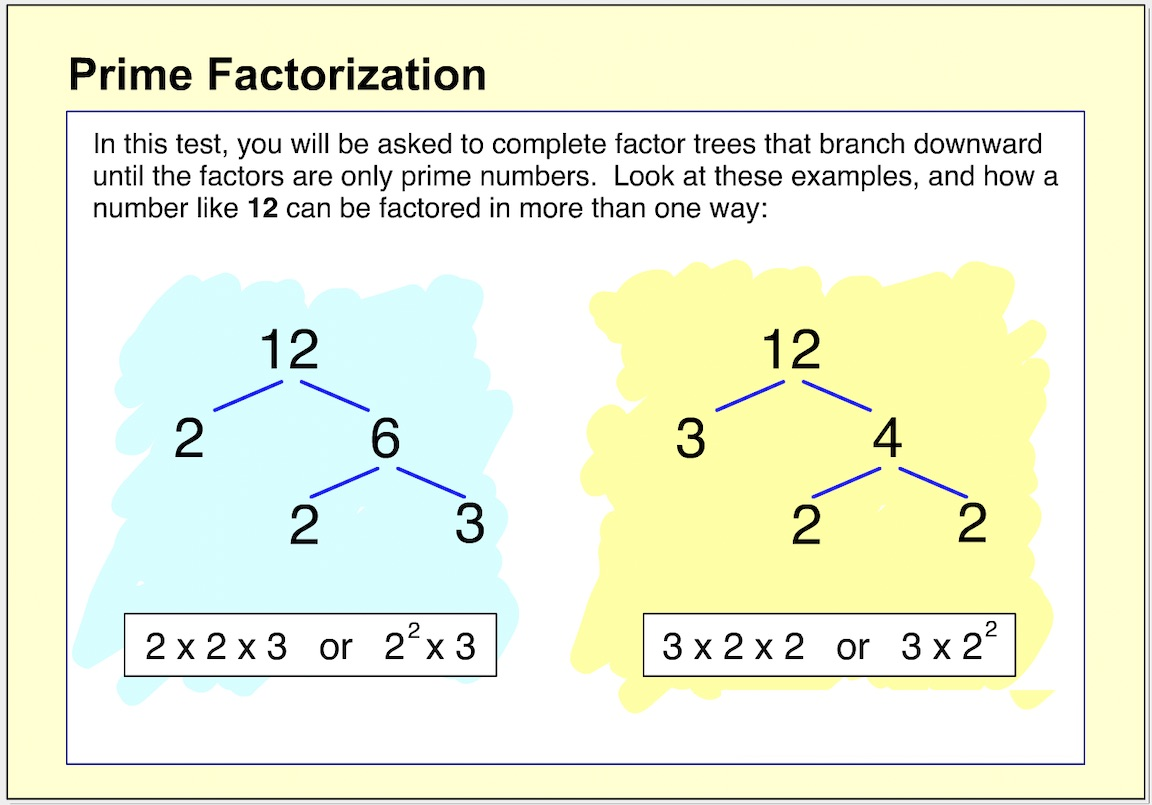Prime Factorization Help Slide 2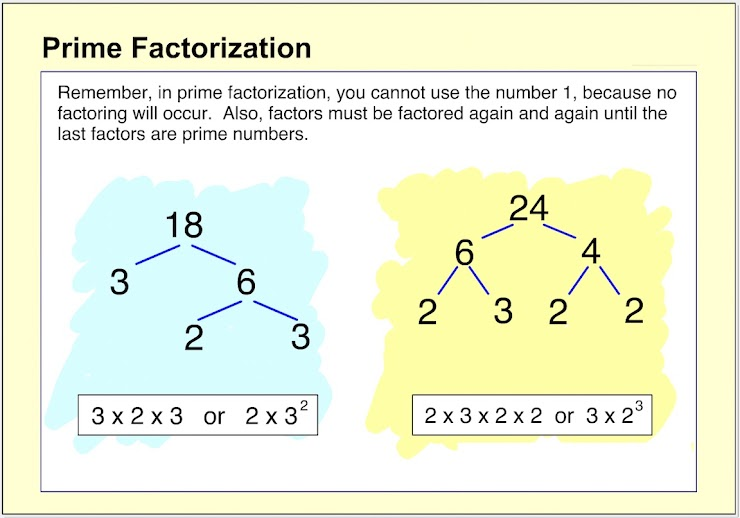Prime Factorization Help Slide 3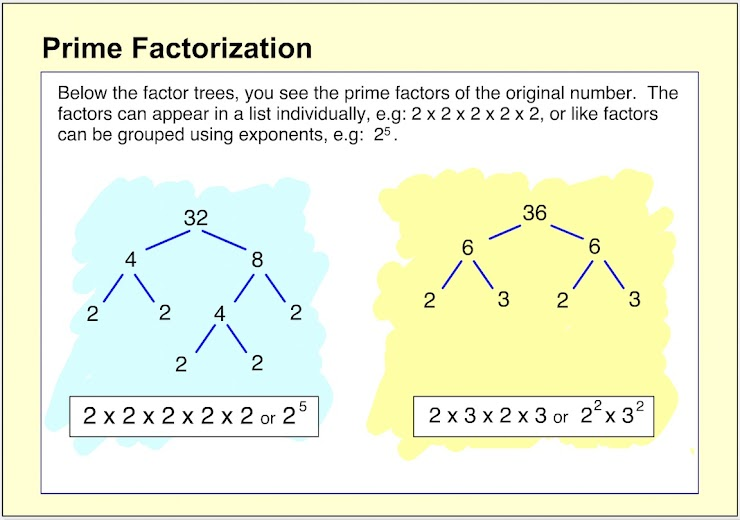Prime Factorization Help Slide 4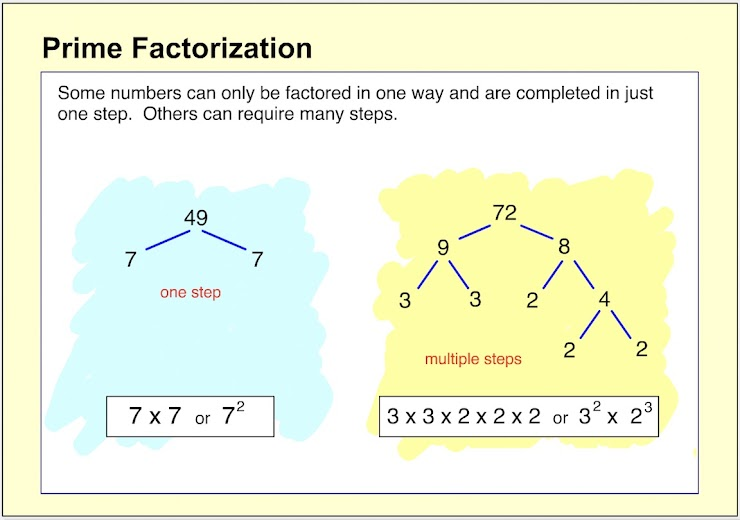Prime Factorization Help Slide 5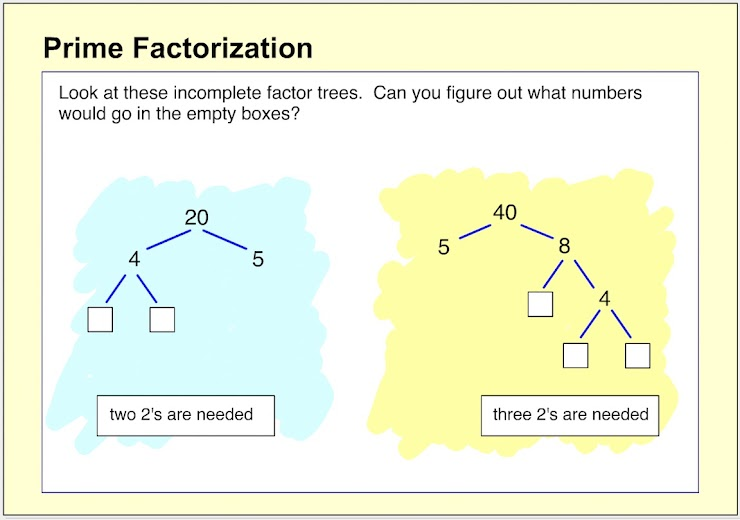1.  What number would go in the white box to complete this factor tree? *
1 point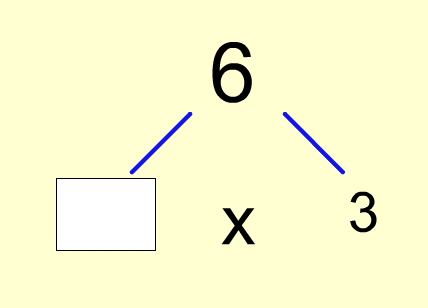2. What number would go in the white box to complete this factor tree? *
1 point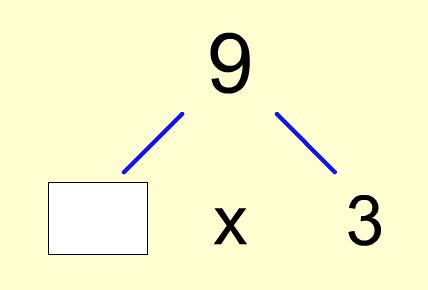3. What number would go in the white box to complete this factor tree? (Enter your answer on the line below.) *
1 point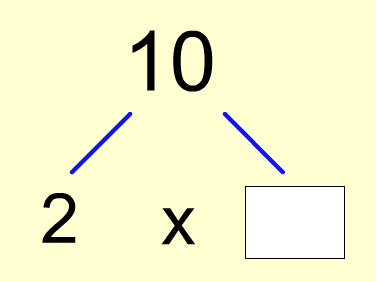4. What number would go in the white box to complete this factor tree? (Enter your answer on the line below.) *
1 point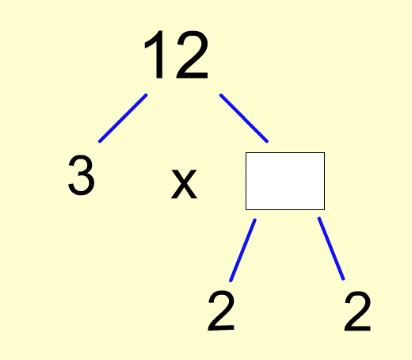5.  What number would go in the white box to complete this factor tree? (Enter your answer on the line below.) *
1 point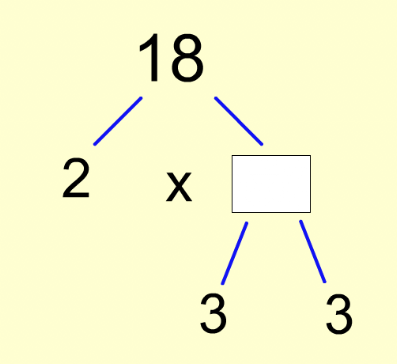6.  What number would go in the white box to complete this factor tree? *
1 point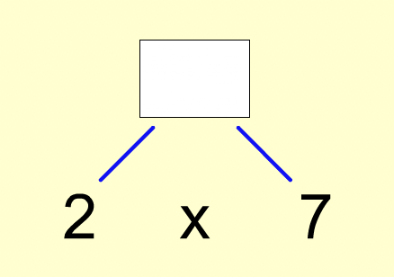7.  What same number would go in each of the white boxes to complete this factor tree? *
1 point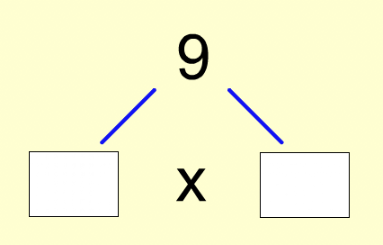8.  What two numbers would go in the two white boxes to complete this factor tree? *
1 point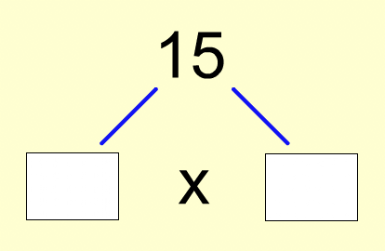9.  Which factor tree is -not- finished? *
1 point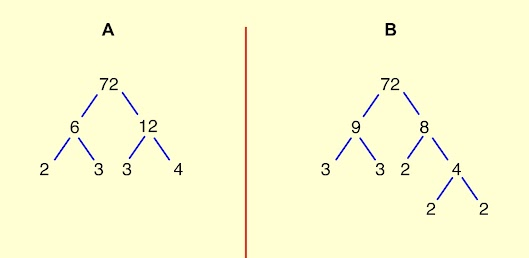10.  Which factor tree is -not- finished? *
1 point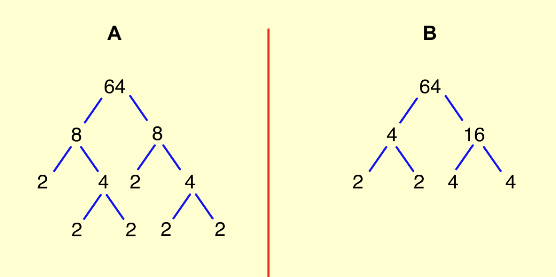Submit
Clear form